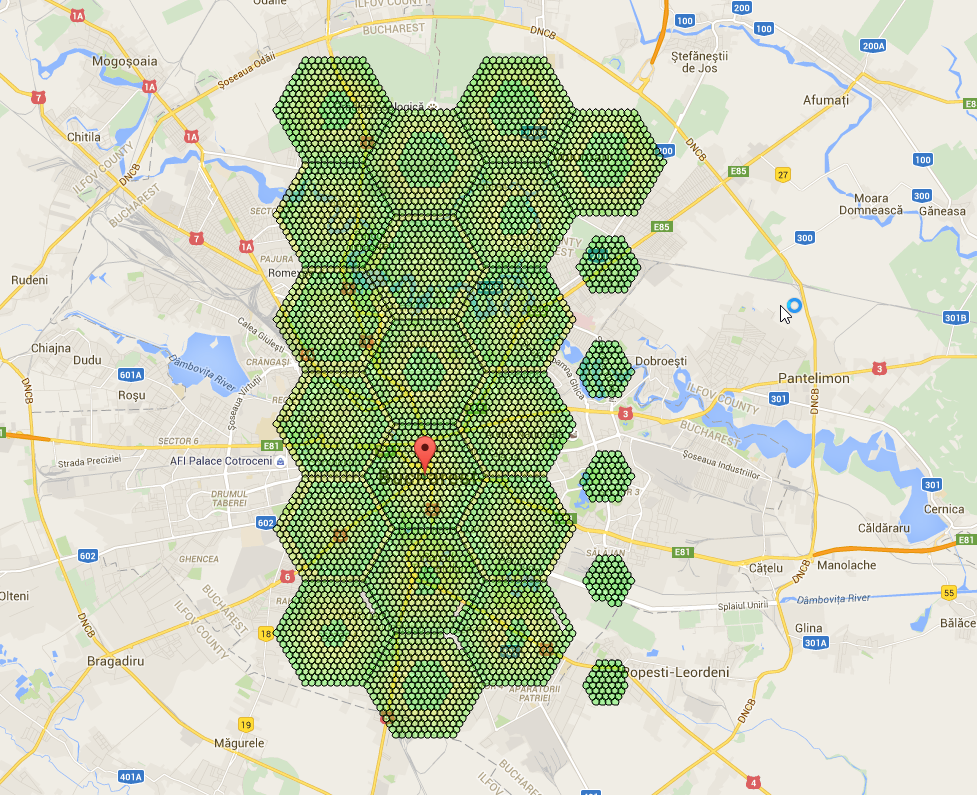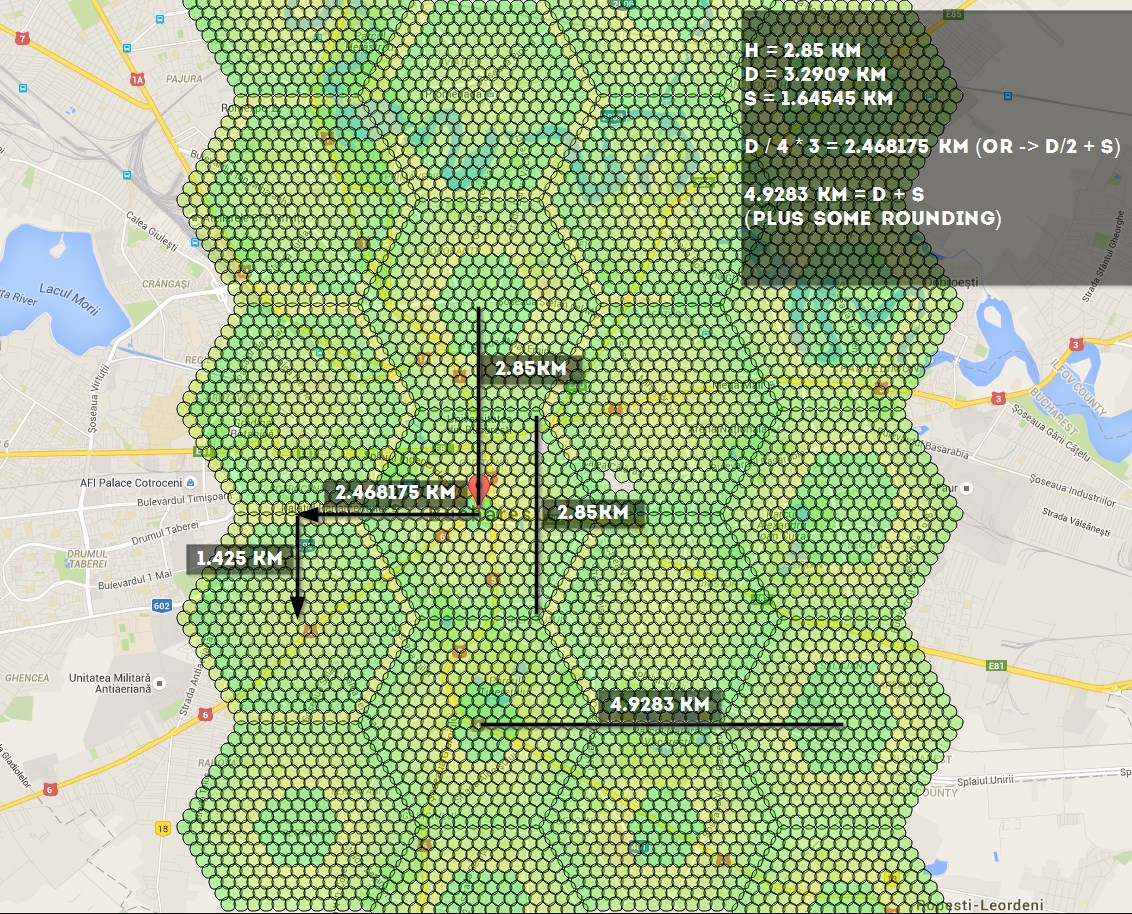# Workers¶

(work in progress)

So, you want to do this:if using -st 10, these are the numbers you should know: 4.9283 km, 1.425 km, 2.468175 km, 2.85 km - you can use the distances to calculate coords here http://www.sunearthtools.com/tools/distance.php

Distances:Link to figure out how to link all the hexagons together: http://goo.gl/X81fZq

contributed by olathurl from discord

## Here’s a half-arsed implementation of reverse Haversine for you to finish off¶

It outputs the commands for scanning, and then prints the command to start the web server

```# coding: utf8
import math

# Earth’s radius, sphere
R = 6378.1

cmd = "python runserver.py -ns -l '{lat} {lon}' --port {port} -u {username} -st 10 -p {password} &"
cmd_final = "python runserver.py -l '{lat} {lon}' -u {username} -st 10 -p {password} -st 10 -t 0 &"

# starting point
sham = (-33.8961, 151.1543)
lat = sham
lon = sham

# offsets in km
d = 2.85
brng = 90

# scan initial location
port = 6001

for i in range(5):
port = port + 1
# // Coordinate offsets in radians
lat1 = math.radians(lat)  # Current lat point converted to radians
lon1 = math.radians(lon)  # Current long point converted to radians

lat2 = math.asin(math.sin(lat1) * math.cos(d / R) +
math.cos(lat1) * math.sin(d / R) * math.cos(brng))

lon2 = lon1 + math.atan2(math.sin(brng) * math.sin(d / R) * math.cos(lat1),
math.cos(d / R) - math.sin(lat1) * math.sin(lat2))

lat2 = math.degrees(lat2)
lon2 = math.degrees(lon2)